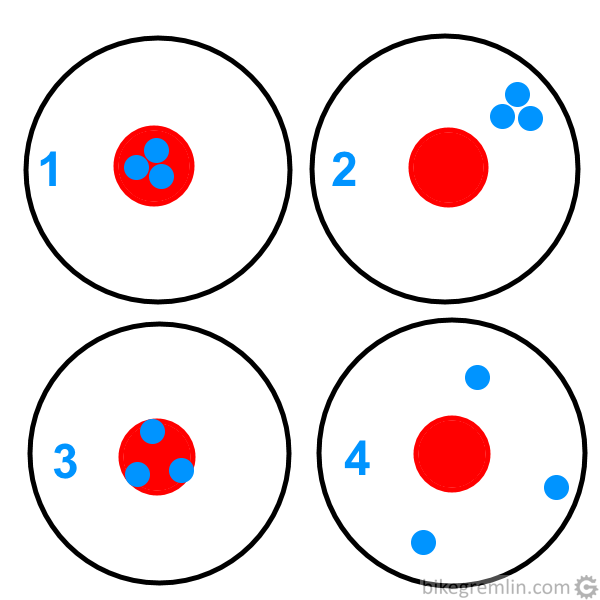Home » Basics » Basics explained » Accurate vs Precise

# Accurate vs Precise

In every day speech, terms “accurate” and “precise” are used interchangeably, as synonyms. In mechanics, especially in measurement, the meaning of these two terms differs. Since I am and will be using these terms, it would be wise to clearly define them.

Before giving any (boring) definitions, I think a picture will speak a lot more clearly:1: Accurate and precise 2: Inaccurate and precise 3: Accurate and imprecise 4: Inaccurate and imprecise

Definitions, before an explanation in “plain English”:

• Accuracy: how close is the value gained by measuring to the real value.
• Precision: how close are the results of each repeated measurement (of the same object) close to each other.

Let us take weight (mass) measurement for an example. Say we are measuring a 70 kg heavy cyclist, using 4 different scales and repeating the measurement 3 times in a row with each scale. Measurement results are shown in table below:

• Scale 1 is both accurate and precise.
• Scale 2 is very precise, but not accurate.
• Scale 3 is pretty accurate, but not very precise.
• Scale 4 is neither accurate, nor precise.

As can be seen in this explanation, terms accurate and precise are connected and somewhat similar, but not exactly the same.